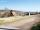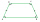Trapezium 2

Trapezium has an area of 24 square cms. How many different trapeziums can be formed ?

Result

n = (Correct answer is: inf)Solution:Leave us a comment of example and its solution (i.e. if it is still somewhat unclear...):Be the first to comment!Next similar examples:

1. TrapeziumThe area of trapezium is 35cm2 find its altitude if the bases are 6cm and 8cm.
2. A trapezoidA trapezoid 75 ft wide on top 85 ft on the bottom, the height is 120 ft. What is its area in the square yds?
3. Area of ditchHow great content area will have a section of trapezoidal ditch with a width of 1.6 meters above and below 0.57 meters? The depth of the ditch is 2.08 meters.
4. Parcelparcel has a rectangular shape of a trapezoid with bases 12 m and 10 m and a height 8 m. On parcel was built object with a footprint an isosceles triangle shape with side 4 m and height three-quarters of a meter. What is the area of unbuild parcel?
5. AcreagePlot has a diamond shape, its side is 25.6 m long and the distance of the opposite sides is 22.2 meters. Calculate its acreage.
6. CableCable consists of 8 strands, each strand consists of 12 wires with diameter d = 0.5 mm. Calculate the cross-section of the cable.
7. Isosceles trapezoid v3In an isosceles trapezoid ABCD is the size of the angle β = 81° Determine size of angles α, γ and δ.
8. Percents - easyHow many percent is 432 out of 434?
9. Sales offGoods is worth € 70 and the price of goods fell two weeks in a row by 10%. How many % decreased overall?
10. Apples 2James has 13 apples. He has 30 percent more apples than Sam. How many apples has Sam?
11. NumberWhat number is 20 % smaller than the number 198?
12. EquationSolve the equation: 1/2-2/8 = 1/10; Write the result as a decimal number.
13. MO 2016 Numerical axisCat's school use a special numerical axis. The distance between the numbers 1 and 2 is 1 cm, the distance between the numbers 2 and 3 is 3 cm, between the numbers 3 and 4 is 5 cm and so on, the distance between the next pair of natural numbers is always in
14. The percentages in practiceIf every tenth apple on the tree is rotten it can be expressed by percentages: 10% of the apples on the tree is rotten. Tell percent using the following information: a. in June rained 6 days b, increase worker pay 500 euros to 50 euros c, grabbed 21 fro
15. Unknown numberIdentify unknown number which 1/5 is 40 greater than one tenth of that number.
16. ClassIn a class are 32 pupils. Of these are 8 boys. What percentage of girls are in the class?
17. TVsProduction of television sets increased from 3,500 units to 4,200 units. Calculate the percentage of production increase.